Solving for x
Exponents
Order of Operations
Distributive Property
100

4x = 16

4

100

When you see the following expression, what is the big number called and what is the little number on top of it called?

24

100

Simplify the following expression: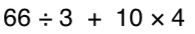100

-4 + -5

-9100

8(4+2) = ?

48

200

x2 = 64

8

200

How can you rewrite 9in expanded form?

9 x 9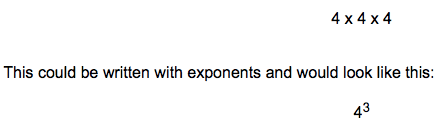200

Simplify the following expression: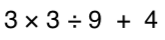200

14 + -12

2

https://www.khanacademy.org/math/arithmetic/arith-review-negative-numbers/arith-review-add-negatives-intro/v/adding-negative-numbers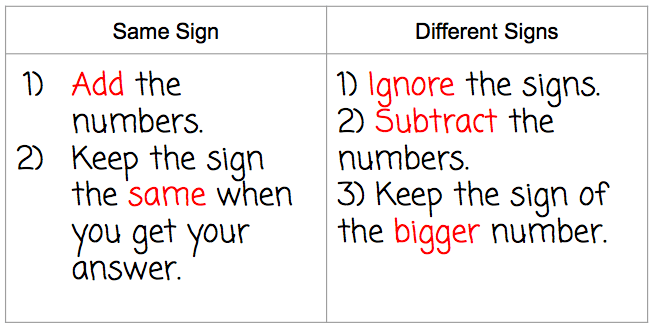200

5(x-3)

5x-15

300

2(x+4) = 10

1

300

How can you rewrite the following in exponential form?

7 x 7 x 7 x 7 x 7

75300

3 + 42 * (3 + 2)

83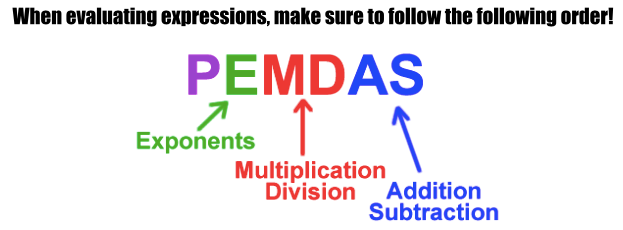300

-10 + 15

5300

7+9(2x-3)

18x-20

400

4(x-3) = 5(x+2)

-22

400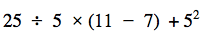45400

-2 + -3 + 5

0

https://www.khanacademy.org/math/arithmetic/arith-review-negative-numbers/arith-review-add-negatives-intro/v/adding-negative-numbers400

-3(-x-10)

3x+30

500

16x2=64

2
500

What is the value of:

23 + 32 - 42

50030500

6 + (-2 + -5)

-1

500

Solve for x

-4y + 2x + 28 = 0

x = 2y - 14

Click to zoom